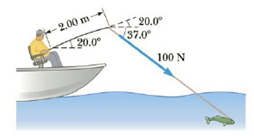Chapter 8, Problem 3P

Chapter
Section
Textbook Problem

The fishing pole in Figure P8.3 makes an angle of 20.0° with the horizontal. What is the magnitude of the torque exerted by the fish about an axis perpendicular to the page and passing through the angler’s hand if the fish pulls on the fishing line with a force F → =  1 .00  ×  10 2 N at an angle 37.0° below the horizontal? The force is applied at a point 2.00 m from the angler’s hands.Figure P8.3

To determine
The magnitude of torque exerted by the fish about an axis perpendicular to the page and passing through the angler’s hand.

Explanation

Figure shows the torque of fishing pole due to the pulling force of a fish.

The force exerted by the fish can be resolved as horizontal and vertical components of force and the horizontal component of force is parallel to the fishing pole, so the torque by this force component becomes zero (since τ=r×F=0 ) but the vertical component of force gives its contribution to the torque that is τ=r×F=rFsinθ0 .

Given info: The length of fishing pole is 2.00m , the force due to pulling of fish is 1.00×102N , the angle of the force with respect to horizontal is 37.0° , and the angle of fishing pole with respect to horizontal is 20.0° .

The formula for the magnitude of the torque is,

τ=rFsin(θ1+θ2)

• r is length of the fishing pole.
• F is the force due to pulling of fish.
• θ1 is the angle of fishing pole with respect to horizontal.
• θ2 is the angle of force due to pulling fish with respect to horizontal.

Substitute 2

Still sussing out bartleby?

Check out a sample textbook solution.

See a sample solution

The Solution to Your Study Problems

Bartleby provides explanations to thousands of textbook problems written by our experts, many with advanced degrees!

Get Started

Find more solutions based on key concepts